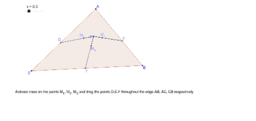•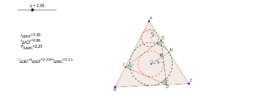### The sum of the lengths of the radiuses of circles inscribed in an equilateral triangle

Activity

•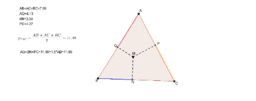### The sum of the lengths of segments on the sides of an equilateral triangle

Activity

•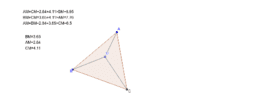### Segments in an equilateral triangle

Activity

•### Segments in an equilateral triangle

Activity

•### Segments in an equilateral triangle

Activity

•### Segments in an equilateral triangle

Activity

•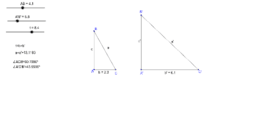### The minimum value of the sum of the lengths of the hypotenus

Activity

•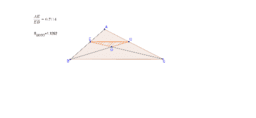### The maximum area of some triangle(stage b).

Activity

•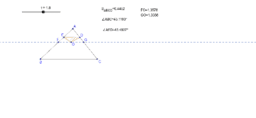### The maximum area of some triangle(stage a).

Activity

•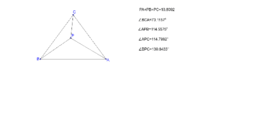### : The sum of the distances is minimal(stage b).

Activity

•### The sum of the distances is minimal(stag a)

Activity

•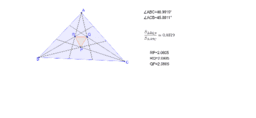### Morley triangle with a maximum relative area.

Activity

•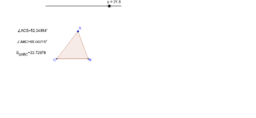### Triangle with a maximum area

Activity

•### The loci formed by the intersection point of the angle bisec

Activity

•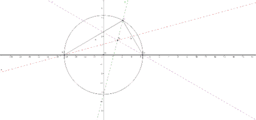### The loci formed by the intersection point of the angle bisec

Activity

•### An applet for demonstrate the loci formed by the intersectio

Activity

•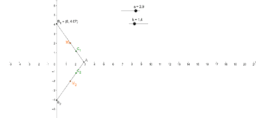### An applet for demonstrate the loci formed by a point on slid

Activity

•### The loci formed by the intersection point of the medians of

Activity

•### The loci formed by the intersection point of the angle bisec

Activity

•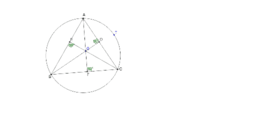### The loci formed by the intersection point of the altitudes

Activity

•### Applet for demonstrating Van Aubel's theorem for quadrilater

Activity

•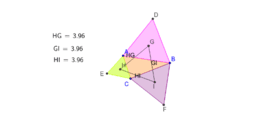### Applet for demonstrating Napoleon's theorem for triangle

Activity

•### : Applet for demonstrating Steiner’s theorem for trapezoid

Activity

•